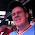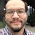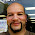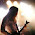Tuesday, December 17, 2013

HP Prime: CAS Commands in Home Mode

(Common) CAS Commands in Home Mode
(udpated 4/17/2014)

Two things to aware of:

1. Any command from the CAS mode to be used in the Home Mode, regardless of entry, must have a "CAS." prefix. Examples include CAS.diff, CAS.simplify, etc.
2. Algebraic (symbolic) objects need single quotes surrounding them. They can be called by pressing Shift+Parenthesis Key ().
3. Not all CAS commands work as expected in Home and Programming. My focus is on the Home mode.

Simplify: CAS, 1, 1

Syntax:
Non-RPN: CAS.simplify('expression')
RPN: Not recommended - as it will numerically evaluate the expression

Expand: CAS, 1, 3

Syntax: CAS.expand('expression')
RPN: Not recommended - as it will numerically evaluate the expression

Example: CAS.expand('(X+3)^2') returns X^2+6*X+9

Factor
There are two commands for factoring:

Polynomial: CAS.Factor: CAS, 1, 4
Non-RPN Syntax: CAS.factor('expression')
Example: CAS.Factor('X^2-5*X+4') returns (X-4)*(X-1)

Integer: CAS.ifactor: CAS, 5, 2
Non-RPN Syntax: CAS.ifactor(integer)
Example: CAS.ifactor(186) returns 2*3*31

RPN Syntax:
1: integer
CAS.ifactor(1), press Enter

Differentiation: CAS, 2, 1
Non-RPN Syntax: CAS.diff('expression')

Simplification may be needed. So call up CAS.simplify, put up single quotes using Shift+Parenthesis Key, key up to the expression, press Copy (soft key), then press Enter

Example:
CAS.diff('COS(X)*SIN(X)') returns -SIN(X)*SIN(X)+COS(X)*COS(X)
CAS.simplify('-SIN(X)*SIN(X)+COS(X)*COS(X)') returns 2*COS(X)^2-1

RPN Syntax:
1: 'f(X)'
CAS.diff(1), press Enter

Update (4/17/2014):  If the above does not work, try this syntax:

CAS.diff('expression', 'variable')

Example:  CAS.diff('COS(X)*SIN(X)', 'X') returns  -SIN(X)*SIN(X)+COS(X)*COS(X)

RPN:
2:  'expression'
1:  'variable'
CAS.diff(2), press enter

Integration: CAS, 2, 2
Non-RPN Syntax: CAS.int('expression')

Simplification may be needed. So call up CAS.simplify, put up single quotes using Shift+Parenthesis Key, key up to the expression, press Copy (soft key), then press Enter

Example:
CAS.int('2*e^(4*X)') returns 2*e^(4*X)/4
CAS.simplify('2*e^(4*X)/4') returns e^(4*X)/2

Not recommended for RPN Entry

Update (4/17/2014):  If the above syntax does not work, try:

CAS.int('expression', 'variable')

Example:
CAS.int('2*X*e^(4*X)','X') returns 2*(4*X-1)/16*e^(4*X)
CAS.simplify('2*(4*X-1)/16*e^(4*X)') returns (4*X*e^(4*X) - e^(4*X))/8

Summation (Σ): CAS, 2, 5
Non-RPN Syntax: CAS.sum('expression', 'variable', start, end)
Example: CAS.sum('N^3','N',1,9) returns 2025

RPN Syntax:
4: 'expression'
3: 'variable'
2: start
1: end
CAS.sum(4) press Enter

Note: Start and End must be numerical limits.

Solving

I recommend zeros and cZeros, not solve and cSolve. The syntax is pretty much the same.

Access:
zeros: CAS, 3, 2
cZeros: CAS, 3, 4

If the equation is f(X) and we are solving for X:

Non-RPN Syntax: CAS.zeros('f(X)')

RPN Syntax:
1: 'f(X)'
CAS.zeros(1), press Enter

Example:
CAS.zeros('X^3-6') returns [1.81712059283]

If you are solving for variables other than X:

Non-RPN Syntax: CAS.zeros('expression', 'variable')

RPN Syntax:
2: 'expression'
1: 'variable'
CAS.zeros(2), press Enter

Got to include the variable!

Example:
CAS.zeros('R^3-6') returns [].
CAS.zeros('R^3-6','R') returns [1.81712059293]

Hope this helps and we will cover more of this subject in the future.

Eddie

This blog is property of Edward Shore. 2013

1.Thank you Eddie for showing how to prepare and execute some CAS commands in the Home mode.

However, after keying in most of these on the calculator, it felt slow and error prone versus just using CAS mode.

RPN (where CAS supported) seemed almost as quick as CAS mode.

1.I wish the RPN mode wasn't so limited in CAS abilities.

2.Help: As advised, in home mode I enter CAS.diff('COS(X)*SIN(X)') but the calculator returns "0" instead of the differentiated function. This is typical of my results. My version info is 2013 11 25 (5447), CAS version 1.1.0-27

1.Try CAS.diff('COS(X)*SIN(X)','X') Hopefully that will work with Ver. 5447.

Since this blog entry was done with an earlier software version, I may be looking at updating this entry. Thanks,

Eddie

3.Thank for you. That worked beautifully. I might add that "Complex" needs to be unchecked to get the result you show. I'm a new owner going through painful acclimation. Love your blog. JFS.

1.Thanks James!

Eddie

4.Thanks Eddie for your insight. I was playing around with my new HP Prime and thought I would tackle something benign like Newton's method. I'm just not getting the syntax to call CAS.diff on my function defined as F1(x) in the Function Symbolic View for each of the iterations. I've tried "f := CAS.diff('F1','X');" but to no avail. Do you have ideas on this?

1.Sorry for getting back to you so late.

On the Function App, Symb View, what you want is to use the Derivative template. The Derivative Template on the top row, 4th column on the template touch menu.

Set up: F1(X) = (d f(T))/(d T = X)

d is the derivative symbol
T is a dummy variable

Example: (d T^3)/(d T = X)

Video on calculating derivatives on the HP Prime (general): https://www.youtube.com/watch?v=LXwLiPlKO2M&list=UU25vsJdvgf_doIgE8-Lu7Xw

Hope this helps, again, apologizes for being super late. :(

Eddie

5.Hi!
I just went from 50g to prime and i struggle a little with pretty simple things :)
Could you please post an example on how to solve for zeros in Home with all keystrokes included? I have mine set to RPN mode

1.With X as a variable: Find the root of 2*X-3

Enter '2*X-3':
[Shift] [ ( ) ] (''), 2 [ * ] [Alpha] [ * ] (X)[ - ] 3 [Enter]

Call up zeros: CAS.zeros(1)
[Toolbox (2nd key from left on the top row of white keys)], 3 for Solve, 2 for zeros, type 1, [Enter]

Result: [ 1.5 ]

It's pretty much the same situation for variables other than 0 except the variable has to be specified. Example: Find the root of N^2-4.

Typing 'N^2-4':
[Shift] [ () ] (''), [Alpha] [ () ] (N), [x^2], [ - ], 4 , [Enter]

Typing 'N' (specify the variable to solve)
[Shift] [ () ] (''), [Alpha] [ () ] (N), [Enter]

Calling up Zeros. This type we want 2 arguments: CAS.zeros(2)
[Toolbox (2nd key from left on the top row of white keys)], 3 for Solve, 2 for zeros, type 2, [Enter]

Result: [ 2, -2 ]

Hope that helps,

Eddie

2.Thanks! Turns out i didn't figure out that i had to put the 1 in the parentheses.. But when i use CAS commands in HOME view I have to remove "CAS." from the command to avoid error, any idea why?

6.I think this is one of the most significant information for me.And i’m glad reading your article.But should remark on some general things, the web site style is perfect,the articles is really great : D. Good job…
IWIN89

7.Likewise in the online study hall, understudies can concentrate on learning math legitimately, since they don't need to stress over staying aware of their schoolmates or the instructor going excessively quick. math

HP 12C: Finding Two Factors of an Integer

HP 12C:  Finding Two Factors of an Integer  Introduction This program finds two factors of the integer N, where one of the factors ...